# Tourism Demand Modeling and Forecasting with PROC VARMAX

Contents | SAS Program

# Overview

Tourism demand modeling and forecasting are very important for tourism-related business decision making. This example illustrates modeling tourism demand using the VARMAX procedure.

# Analysis

Tourism demand can be measured in terms of

• number of tourist visits from an origin country to a destination country
• tourist expenditure by visitors from the origin country in the destination country
• tourist nights spent by visitors in the destination country

Generally, the explanatory variables for tourism demand include origin country income, destination country tourism prices, substitute destination country tourism prices, tastes, etc. Empirical studies usually use living costs for tourists in the destination as the tourism price. Various demand models can be used to estimate and forecast tourism demand.

This example considers modeling tourism demand in a vector autoregressive (VAR) framework. The goal is to forecast the number of holidays in Spain taken by United Kingdom (U.K.) residents using annual tourism data from 1966 to 1994 obtained from Song and Witt (2000). The variables in the data set are described in the following table:

 SAS Variable Name Description VSP The number of holidays in Spain taken by U.K. residents PDI U.K. real personal disposable income PUK The implicit deflater of U.K. consumer expenditure EXSP Exchange rate index of Spanish pesetas against the US dollar EXUK Exchange rate index of U.K. pound against the U.S. dollar POP U.K. population CPISP The consumer price index in Spain

The log of the number of per capita holiday visits to Spain by U.K. residents is plotted in Figure 1 using the following code:

   data tourism ;
set sashelp.tourism;
lpvsp = log(vsp);
lppdi = log(pdi);
lrcsp = log( (cpisp/exsp)/(puk/exuk) );
label lpvsp='log(per capita holiday visits to Spain)'
lppdi='log(per capita disposable income)'
lrcsp='log(living costs in Spain relative to
UK living costs adjusted by the exchange rate)';
run;


    proc gplot data = tourism ;
plot lpvsp*year / cframe = ligr vaxis = axis1 haxis = axis2 ;
title 'Log of Per Capita Holiday Visits to Spain' ;
symbol c = blue i = join v = star ;
axis1 label = (angle=90 'log(per capita visits to Spain)') ;
axis2 label = ('Year') ;
run ;
quit ;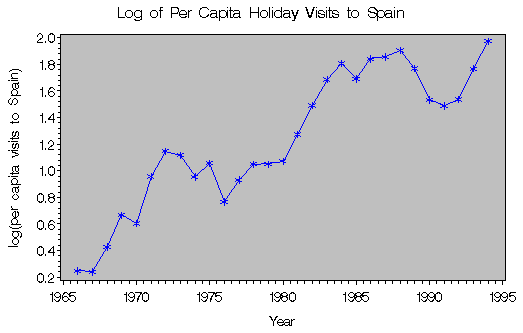Figure 1: Log of Per Capita Holiday Visits to Spain

The VAR model of order one can be expressed as follows:
where Yt is a k by 1 observation vector,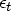is a k by 1 white noise vector, C is a k by 1 vector of parameters, and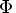is a k by k matrix of first order autoregressive parameters.

In the tourism model, the vector Yt is (LPVSPt,LPPDIt,LRCSPt), where LPVSP denotes a log of the number of holidays in Spain taken by U.K. residents, LPPDI denotes a log of U.K. real personal disposable income, and LRCSP denotes a log of living costs in Spain relative to the U.K. adjusted by the exchange rate. LRCSP is obtained by the relation

LRCSP = ln([(cpisp/exsp)/(puk/exuk)])

where cpisp is the consumer price index in Spain, exsp is the exchange rate index of Spanish pesetas against the U.S. dollar, puk is the implicit deflater of U.K. consumer expenditure, and exuk is the exchange rate index of the U.K. pound against the U.S. dollar.

The tourism model can be written as
You can use the VARMAX procedure to fit this VAR(1) model. First, state the variable names in the MODEL statement. Then use the P= option in the MODEL statement to specify the order of the VAR processes.

To determine the appropriate order of the VAR processes to be used, you can look at the partial autoregressive matrices, partial correlation matrices, or partial canonical matrices. These matrices have the cutoff property for a VAR(p) model at lag = p. Using the PRINT=(PARCOEF PCORR PCANCORR) option enables you to print out the necessary matrices, as shown in the following code:

   proc varmax data=tourism;
id year interval=year;
model lpvsp lppdi lrcsp / p=1 print=(parcoef pcorr pcancorr) lagmax=6 ;
run ;


The VARMAX Procedure

Schematic Representation of
Partial Autoregression
Variable/Lag 1 2 3 4 5 6
lpvsp ... ... ... ... ... ...
lppdi .+. ... ... ... ... ...
lrcsp ..+ ... ... ..- ... ...

Figure 2: Partial Autoregressive Coefficients

Figure 2 shows that the model can be obtained by an AR order p=1 since partial autoregression matrices are insignificant after lag 1 with respect to two standard errors.

Schematic Representation of
Partial Cross Correlations
Variable/Lag 1 2 3 4 5 6
lpvsp +.. ... ... ... ... ...
lppdi .+. ... ... ... ... ...
lrcsp ..+ ... ... ..- ... ...

Figure 3: Partial Correlations

The partial cross-correlation matrices in Figure 3 are insignificant after lag 1 with respect to two standard errors. This indicates that an AR order of p=1 can be an appropriate choice.

Partial Canonical Correlations
Lag Correlation1 Correlation2 Correlation3 DF Chi-Square Pr > ChiSq
1 0.91597 0.79703 0.49657 9 48.18 <.0001
2 0.36954 0.22093 0.12404 9 5.42 0.7962
3 0.37745 0.28020 0.02363 9 5.76 0.7637
4 0.60568 0.25861 0.03195 9 10.87 0.2848
5 0.57268 0.28654 0.07127 9 9.96 0.3535
6 0.51369 0.16498 0.04311 9 6.74 0.6644

Figure 4: Partial Canonical Correlations

Figure 4 shows that the partial canonical correlations between yt and yt-p are {0.91597, 0.79709}, {0.36957, 0.22090}, {0.37744, 0.28023} for lags p=1 to 3. After lag p=1, the partial canonical correlations are insignificant with respect to the 0.05 significance level, indicating than an AR order of p=1 would be an appropriate choice.

Since the results show that the partial autoregressive matrices, partial cross correlation matrices, and partial canonical correlation matrices are all insignificant after lag 1 with respect to two standard errors, a VAR(1) model is appropriate. The parameter estimates of the VAR(1) model are shown in Figure 5.

Model Parameter Estimates
Equation Parameter Estimate Standard
Error
t Value Pr > |t| Variable
lpvsp CONST1 -8.78015 3.61890 -2.43 0.0231 1
AR1_1_1 0.66753 0.11795 5.66 0.0001 lpvsp(t-1)
AR1_1_2 0.73131 0.29788 2.46 0.0217 lppdi(t-1)
AR1_1_3 -0.76821 0.22902 -3.35 0.0026 lrcsp(t-1)
lppdi CONST2 1.32903 0.63340 2.10 0.0466 1
AR1_2_1 0.05094 0.02064 2.47 0.0211 lpvsp(t-1)
AR1_2_2 0.89084 0.05214 17.09 0.0001 lppdi(t-1)
AR1_2_3 -0.02118 0.04008 -0.53 0.6020 lrcsp(t-1)
lrcsp CONST3 1.44949 2.99679 0.48 0.6330 1
AR1_3_1 0.08103 0.09768 0.83 0.4149 lpvsp(t-1)
AR1_3_2 -0.12599 0.24667 -0.51 0.6142 lppdi(t-1)
AR1_3_3 0.61769 0.18965 3.26 0.0033 lrcsp(t-1)

Figure 5: Parameter Estimates of the VAR(1) Model

Figure 6 plots the residuals obtained from the equation for LPVSP.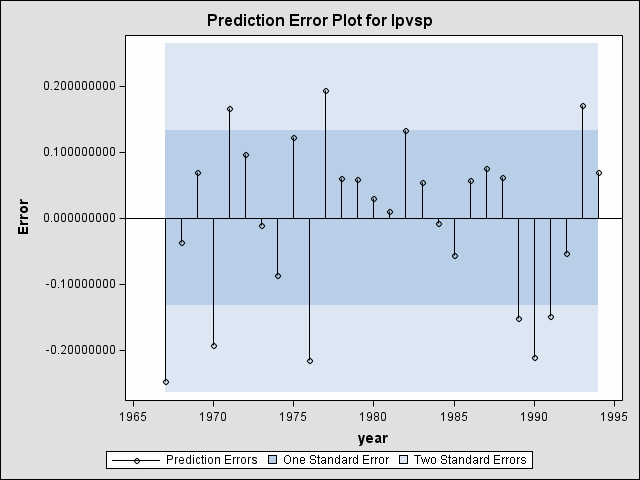Figure 6: Residual Plot of the VAR(1) Model

The preceding residual plot shows that prediction errors from the VAR(1) model are all within two standard errors. This also provides some support for choosing the first order VAR model.

You can also use the CAUSAL statement in the VARMAX procedure to perform a Granger-Causality test on the variables of interest. The GROUP1=(VARIABLES) GROUP2=(VARIABLES) option enables you to specify the variables to be tested. The null hypothesis of the Granger-Causality test is that GROUP1 is influenced by itself, and not by GROUP2. If the test of hypothesis fails to reject the null, the variables in the GROUP1 may be considered as independent variables. The Granger-Causality test results are shown in Figure 7.

   proc varmax data=tourism;
id year interval=year;
model lpvsp lppdi lrcsp / p=1;
/* Test for Causality */
causal group1=(lrcsp) group2=(lpvsp lppdi);
run;


The VARMAX Procedure

Granger-Causality Wald Test
Test DF Chi-Square Pr > ChiSq
1 2 0.99 0.6105

Figure 7: Granger-Causality Test Results

The Granger-Causality test statistics shows that LRCSP is not influenced by LPVSP and LPPDI. Hence, LRCSP should be treated as an exogenous variable, and the following VARX(1) model is appropriate.
where Yt=(LPVSPt,LPPDIt), and Xt=LRCSPt. The VARX(1,1) model can then be expressed as
You can use the MODEL statement in the VARMAX procedure to estimate the VARX(1,1) model. You first specify the dependent variables, then the equality sign, and then the independent variables. You then use the P= option to specify the order of the VAR processes and the XLAG= option to specify the lag order of the exogenous variables. The VARMAX parameter estimates are shown in Figure 8.

   /* Fit the VARX(1,1) model */
proc varmax data=tourism;
id year interval=year;
model lpvsp lppdi = lrcsp / p=1 xlag=1 ;
run;


The VARMAX Procedure

Model Parameter Estimates
Equation Parameter Estimate Standard
Error
t Value Pr > |t| Variable
lpvsp CONST1 -8.24987 3.54014 -2.33 0.0289 1
XL0_1_1 -0.36584 0.23997 -1.52 0.1410 lrcsp(t)
XL1_1_1 -0.54224 0.26773 -2.03 0.0546 lrcsp(t-1)
AR1_1_1 0.69718 0.11646 5.99 0.0001 lpvsp(t-1)
AR1_1_2 0.68522 0.29156 2.35 0.0277 lppdi(t-1)
lppdi CONST2 1.20369 0.59326 2.03 0.0542 1
XL0_2_1 0.08647 0.04021 2.15 0.0423 lrcsp(t)
XL1_2_1 -0.07460 0.04487 -1.66 0.1100 lrcsp(t-1)
AR1_2_1 0.04394 0.01952 2.25 0.0342 lpvsp(t-1)
AR1_2_2 0.90174 0.04886 18.46 0.0001 lppdi(t-1)

Figure 8: Parameter Estimates of the VARX(1,1) Model

The parameter estimates results show that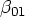and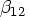are not significant at the 10% significance level, hence, these two parameters can be restricted to be zero. The following residual plot in Figure 9 shows that prediction errors from the VARX(1,1) model are all within two standard errors.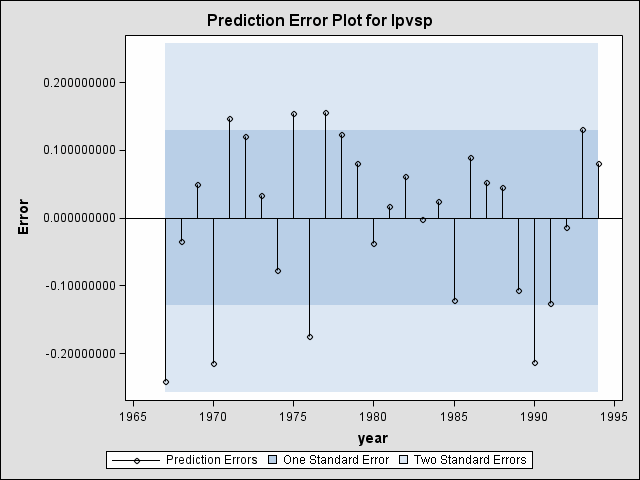Figure 9: Residual Diagnostic for the VARX(1,1) Model

You can use the RESTRICT statement in the VARMAX procedure to restrict parameters in the model. To perform forecasts based on the model estimated, you can use the OUTPUT statement. You need to specify the number of observations to be forecasted with the LEAD= option. The restricted VARX(1,1) model parameter estimates are shown in Figure 10.

   /* Fit the VARX(1,1) model with parameter restriction */
proc varmax data=tourism;
id year interval=year;
model lpvsp lppdi = lrcsp / p=1 xlag=1;
restrict XL(0,1,1) = 0 XL(1,2,1)=0;
run;


The VARMAX Procedure

Model Parameter Estimates
Equation Parameter Estimate Standard
Error
t Value Pr > |t| Variable
lpvsp CONST1 -8.25141 3.51106 -2.35 0.0277 1
XL0_1_1 0.00000 0.00000     lrcsp(t)
XL1_1_1 -0.70675 0.22039 -3.21 0.0039 lrcsp(t-1)
AR1_1_1 0.68178 0.11456 5.95 0.0001 lpvsp(t-1)
AR1_1_2 0.68825 0.28903 2.38 0.0259 lppdi(t-1)
lppdi CONST2 1.73555 0.51150 3.39 0.0025 1
XL0_2_1 0.05806 0.03310 1.75 0.0928 lrcsp(t)
XL1_2_1 0.00000 0.00000     lrcsp(t-1)
AR1_2_1 0.05946 0.01731 3.44 0.0023 lpvsp(t-1)
AR1_2_2 0.85819 0.04218 20.34 0.0001 lppdi(t-1)

Figure 10: Restricted Parameter Estimates

The output also shows the six-year-ahead forecasts on a log of the number of holidays in Spain taken by U.K. residents (LPVSP) and a log of the U.K. real personal disposable income (LPPSI) and their corresponding 95% confidence intervals. These results suggest that on average, U.K. residents are expected to spend about seven days in Spain in 1995. Note that the numbers in the Forecast column in Figure 11 are in logarithm form.

Forecasts
Variable Obs Time Forecast Standard
Error
95% Confidence Limits
lpvsp 30 1995 2.01513 0.13531 1.74993 2.28033
31 1996 2.06105 0.18052 1.70723 2.41487
32 1997 2.10908 0.21402 1.68960 2.52855
33 1998 2.15711 0.23793 1.69077 2.62345
34 1999 2.20441 0.25504 1.70455 2.70427
35 2000 2.25080 0.26781 1.72590 2.77569
lppdi 30 1995 12.91046 0.02370 12.86401 12.95692
31 1996 12.93041 0.03369 12.86438 12.99643
32 1997 12.95011 0.04117 12.86941 13.03080
33 1998 12.96979 0.04751 12.87666 13.06291
34 1999 12.98948 0.05337 12.88487 13.09410
35 2000 13.00917 0.05898 12.89358 13.12477

Figure 11: Six-Year Forecast

Figure 12 plots the forecasts on a log of the number of holidays in Spain taken by U.K. residents and their 95% confidence intervals. The plot shows that future holiday visits to Spain by U.K. residents are expected to increase over the next several years.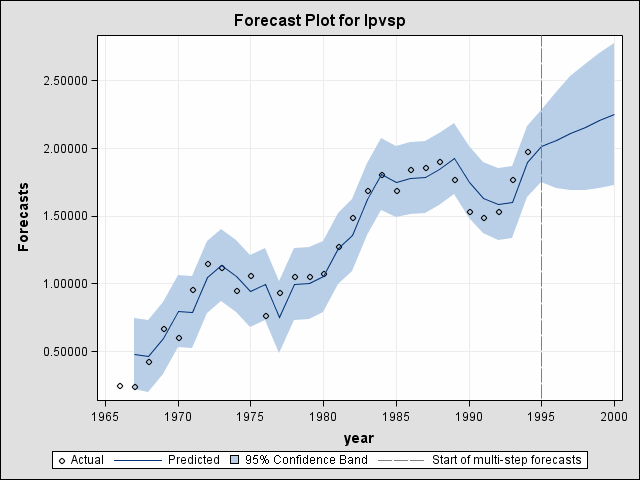Figure 12: Forecasted Log Number of Holidays in Spain

# References

SAS Institute Inc. (1999), SAS/ETS User's Guide, Version 8, Cary, NC: SAS Institute Inc.

SAS Institute Inc. (2004), SAS/ETS User's Guide, Version 9, Cary, NC: SAS Institute Inc.

Song, H. and Witt, S. F. (2000), Tourism Demand Modeling and Forecasting: Modern Econometric Approaches, Oxford, U.K.: Elsevier Science Ltd.